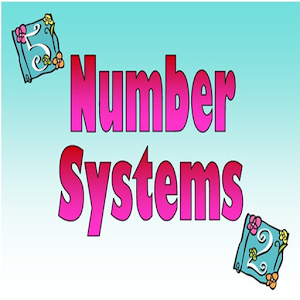# Number System Reference free - Lumos Educational App Store4.31
Price -Free
\$0

#### DESCRIPTION:

The Complete Reference of Numbers System covering the following topics - Natural Numbers - Whole Numbers - Integers - Rational Numbers - Irrational Numbers - Real Numbers - Imaginary Numbers - Complex Numbers - Prime Numbers - Even Odd Numbers - Set Representation - Remainder Concept - Remainder Examples - Negative Remainder Concept - Negative Remainder Examples - Special Cases - Special Cases Examples - Euler Theorem - Euler Theorem Examples - Last 2 digits findings - Divisibility Rules and many more with regular updates . This Number System

#### OVERVIEW:

Number System Reference free is a free educational mobile app By VD.It helps students in grades HS practice the following standards HSN.RN.A.1,HSN.RN.A.2,HSN.RN.B.3.

This page not only allows students and teachers download Number System Reference free but also find engaging Sample Questions, Videos, Pins, Worksheets, Books related to the following topics.

1. HSN.RN.A.1 : Explain how the definition of the meaning of rational exponents follows from extending the properties of integer exponents to those values, allowing for a notation for radicals in terms of rational exponents. For example, we define 51/3 to be the cube root of 5 because we want (51/3)3 = 5(1/3)3 to hold, so (51/3)3 must equal 5.

2. HSN.RN.A.2 : Rewrite expressions involving radicals and rational exponents using the properties of exponents..

3. HSN.RN.B.3 : Explain why the sum or product of two rational numbers is rational; that the sum of a rational number and an irrational number is irrational; and that the product of a nonzero rational number and an irrational number is irrational.

HS

#### STANDARDS:

HSN.RN.A.1
HSN.RN.A.2
HSN.RN.B.3

Developer: VD

Software Version: 2.0.2

Category: Education

### RELATED APPS